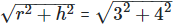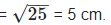Courses

# RD Sharma Solutions -Ex-16.2, Understanding Three Dimensional Shapes, Class 6, Maths Class 6 Notes | EduRev

## Class 6 : RD Sharma Solutions -Ex-16.2, Understanding Three Dimensional Shapes, Class 6, Maths Class 6 Notes | EduRev

The document RD Sharma Solutions -Ex-16.2, Understanding Three Dimensional Shapes, Class 6, Maths Class 6 Notes | EduRev is a part of the Class 6 Course RD Sharma Solutions for Class 6 Mathematics.
All you need of Class 6 at this link: Class 6

Q.1 Give two new examples of each of the for three-dimensional shapes :

(i) Cone (ii) Sphere (iii) Cylinder (iv) Cuboid (v) Pyramid

Sol.1 :

(i) A school bell and a funnel.

(ii) A tennis ball and a model of a globe.

(iii) Drink cans and delivering pipes for a water and gas.

(iv) A match box and brick.

(v) A paper- weight and a tower like the Eiffel tower.

Q.2 What is the shape of :

(i) instrument box

(ii) a brick

(iii) a match box

(iv) a rod- roller

Sol.2 :

(i) My instrument box is in the shape of a cuboid.

(ii) A brick is in the shape of the cuboid.

(iii) A match – box is in the shape of a cuboid.

(iv) A road – roller is in the shape of a cylinder.

(v) A sweet laddoo is shaped like a sphere.

#### OBJECTIVE TYPE QUESTIONS

Q.1. Total number of faces of a cuboid is

(a) 4 (b) 6 (c) 8 (d) 12

Sol. 1 :

(b) 6

Q.2.Total number of edges of a cuboid is

(a) 4 (b) 6 (c) 8 (d) 12

Sol.2 :

(d) 6

Q.3. Number of faces of a cuboid is

(a) 4 (b) 6 (c) 8 (d) 12

Sol.3 :

(c) 8

Q.4. Which one of them is example of cuboid?

(a) a dice (b) a football (c) a gas pipe (d) an ice- cream cone

Sol.4 :

(a) A dice

Q.5. A brick is an example of

(a) cube (b)cuboid (c) prism (d) cylinder

Sol.5 :

(b) Cuboid

Q.6. A gas pipe is an example of

(a)cone (b)a cylinder (c)cube (d)sphere

Sol.6 :

(b) A cylinder

Q.7. If the base radius and height of a right circular cone are 3 cm and 4 cm in lengths, then the slant height is

(a) 5 cm (b) 2 cm (c)25 cm (d)6 cm

Sol.7 :

(a) 5 cm

L =Q.8. The number of faces of a triangular pyramid is

(a) 3 (b) 4 (c) 6 (d) 8

Sol.8 :

(b) 4

A pyramid is called a triangular pyramid if its base is a triangle.

Q.9. The number of edges of a triangular pyramid is

(a) 3 (b) 4 (c) 6 (d) 8

Sol.9 :

(c) 6

Q.10. A tetrahedron is a pyramid whose base is a

(a) triangle (b) square (c) rectangle (d) quadrilateral

Sol.10 :

(a) Triangle

Offer running on EduRev: Apply code STAYHOME200 to get INR 200 off on our premium plan EduRev Infinity!

103 docs

,

,

,

,

,

,

,

,

,

,

,

,

,

,

,

,

,

,

,

,

,

,

,

,

,

,

,

,

,

,

;